# Examples for secondary school students

#### Number of problems found: 2059

• Equation 23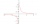Find value of unknown x in equation: x+3/x+1=5 (problem finding x)
• Sequence 3Write the first 5 members of an arithmetic sequence: a4=-35, a11=-105.
• 2nd class variationsFrom how many elements you can create 2450 variations of the second class?
• Goniometric form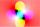Determine goniometric form of a complex number ?.
• Euclid2In right triangle ABC with right angle at C is given side a=27 and height v=12. Calculate the perimeter of the triangle.
• The gems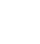The jeweler selects 4 gems for the ring. It has rubies, emeralds and sapphires. How many options does he have?
• Q-Exam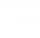If tg α = 9.6, Calculating sin α, cos α, cotg α .
• DiceWe throw 10 times a play dice, what is the probability that the six will fall exactly 4 times?
• WordWhat is the probability that a random word composed of chars E, Y, G, E, R, O, M, T will be the GEOMETRY?
• Lottery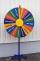The lottery is 60000 elk in which 6200 wins. What is the probability that the purchase of 12 elks won nothing?
• Angle between lines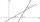Calculate the angle between these two lines: ? ?
• Vector 7Given vector OA(12,16) and vector OB(4,1). Find vector AB and vector |A|.
• Geometric sequence 5About members of geometric sequence we know: ? ? Calculate a1 (first member) and q (common ratio or q-coefficient)
• Root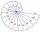Use law of square roots roots: ?
• Playing cards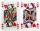How many possible ways are to shuffle 7 playing cards?
• Exponential equationSolve for x: (4^x):0,5=2/64.
• CardsThe player gets 8 cards of 32. What is the probability that it gets a) all 4 aces b) at least 1 ace
• Vans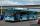In how many ways can 9 shuttle vans line up at the airport?
• CotangentIf the angle α is acute, and cotg α = 1/3. Determine the value of sin α, cos α, tg α.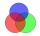78 school students graduate from mathematics or physics. There are three times more students who graduate from mathematics and do not graduate from physics than those who graduate from physics and do not graduate from mathematics. 69 students graduate fro